## ML Aggarwal Class 10 Solutions for ICSE Maths Chapter 9 Arithmetic and Geometric Progressions Ex 9.5

These Solutions are part of ML Aggarwal Class 10 Solutions for ICSE Maths. Here we have given ML Aggarwal Class 10 Solutions for ICSE Maths Chapter 9 Arithmetic and Geometric Progressions Ex 9.5

More Exercises

Question 1.
Find the sum of:
(i) 20 terms of the series 2 + 6 + 18 + …
(ii) 10 terms of series 1 + √3 + 3 + …
(iii) 6 terms of the GP. 1, $$– \frac { 2 }{ 3 }$$ , $$\\ \frac { 4 }{ 9 }$$, …
(iv) 20 terms of the GP. 0.15, 0.015, 0.0015,…
(v) 100 terms of the series 0.7 + 0.07 + 0.007 +…
(vi) 5 terms and n terms of the series $$1+\frac { 2 }{ 3 } +\frac { 4 }{ 9 } +…$$
(vii) n terms of the G.P. √7, √21, 3√7, …
(viii)n terms of the G.P. 1, – a, a², – a³, … (a ≠ – 1)
(ix) n terms of the G.P. x3, x5 , x7, … (x ≠ ±1).
Solution:
(i) 2 + 6 + 18 + … 20 terms
Here, a = 2, r = 3, n = 20, r > 1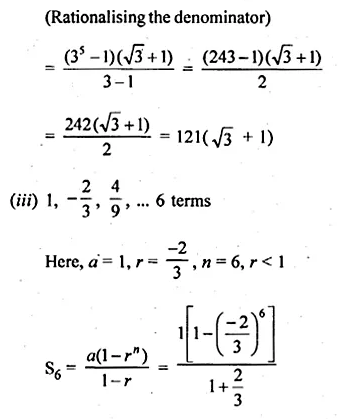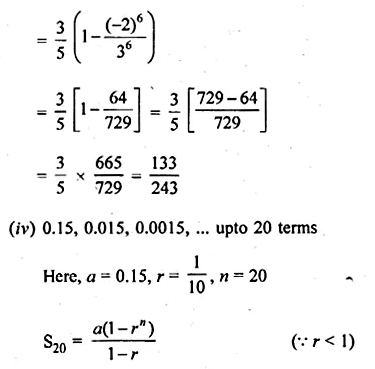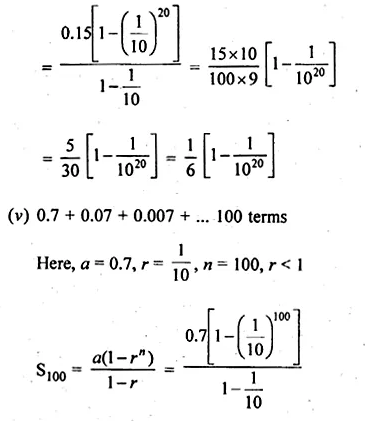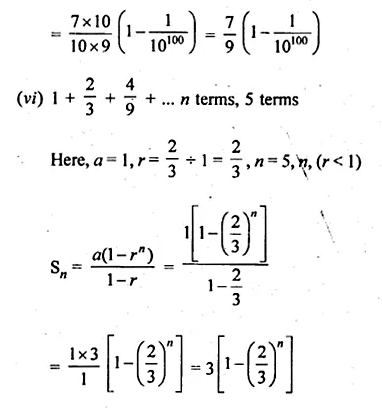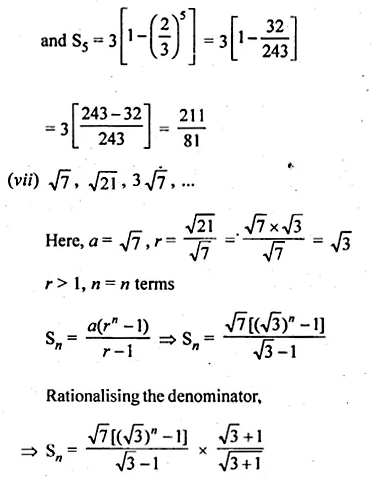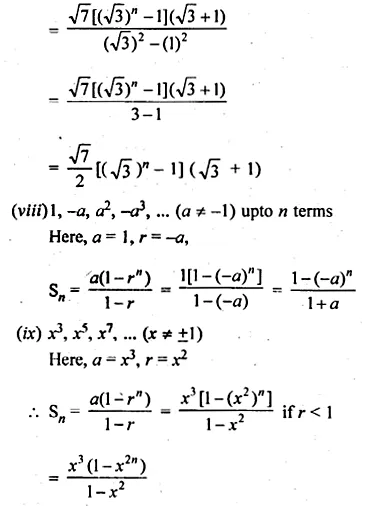Question 2.
Find the sum of the first 10 terms of the geometric series
√2 + √6 + √18 + ….
Solution:
√2 + √6 + √18 + ….
Here, a = √2 , r = √3, r > 1Question 3.
Find the sum of the series 81 – 27 + 9….$$– \frac { 1 }{ 27 }$$
Solution:
Given
81 – 27 + 9….$$– \frac { 1 }{ 27 }$$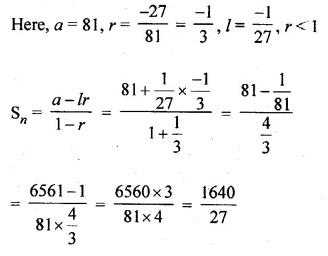Question 4.
The nth term of a G.P. is 128 and the sum of its n terms is 255. If its common ratio is 2, then find its first term.
Solution:
In a G.P.
Tn =128
Sn = 255
r = 2,
Let a be the first term, then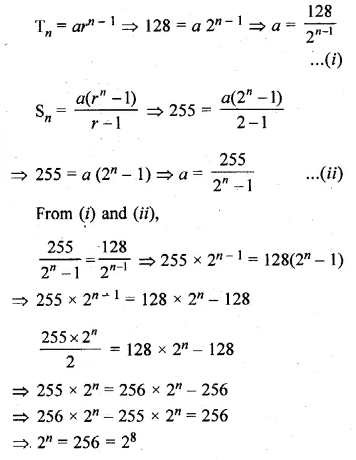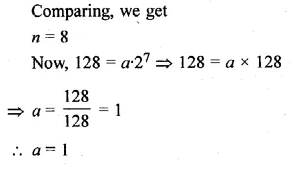Question 5.
If the sum of first six terms of any G.P. is equal to 9 times the sum of the first three terms, then find the common ratio of the G.P.
Solution:
Sum of first 6 terms of a G.P. = 9 x The of first 3 terms
Let a be the first term and r be the common ratioQuestion 6.
A G.P. consists of an even number of terms. If the sum of all the terms is 3 times the sum of the odd terms, then find its common ratio.
Solution:
Let the G.P. be a, ar, ar2, … ar2n – 1
These are 2n in number, which is an even number
A.T.Q.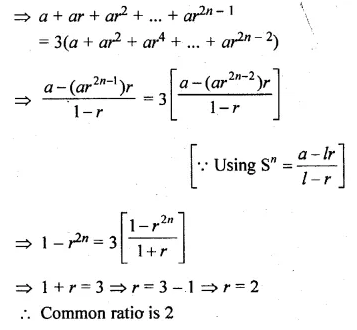Question 7.
(i) How many terms of the G.P. 3, 32, 33, … are needed to give the sum 120?
(ii) How many terms of the G.P. 1, 4, 16, … must be taken to have their sum equal to 341?
Solution:
In G.P.
(i) 3, 32, 33, …
Sum = 120, Here, a = 3, r = $$\frac { { 3 }^{ 2 } }{ 3 }$$ = 3, r > 1Question 8.
How many terms of the GP. 1, √2 > 2, 2 √2 , … are required to give a sum of 1023( √2 + 1)?
Solution:
GP. 1, √2 > 2, 2 √2 , …
Sum = 1023 (√2 + 1)
Here, a = 1, r = √2 . r > 1
Let number of terms be n, then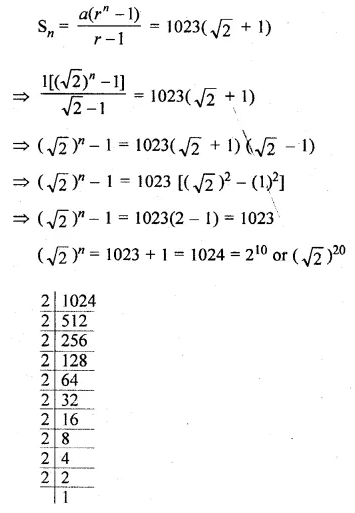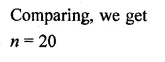Question 9.
How many terms of the $$\frac { 2 }{ 9 } -\frac { 1 }{ 3 } +\frac { 1 }{ 2 } +…$$ will make the sum $$\\ \frac { 55 }{ 72 }$$ ?
Solution:
G.P. is $$\frac { 2 }{ 9 } -\frac { 1 }{ 3 } +\frac { 1 }{ 2 } +…$$
sum $$\\ \frac { 55 }{ 72 }$$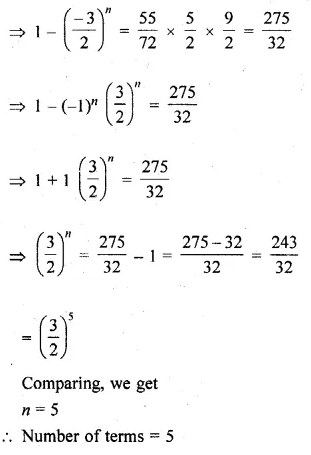Question 10.
The 2nd and 5th terms of a geometric series are $$– \frac { 1 }{ 2 }$$ and sum $$\\ \frac { 1 }{ 16 }$$ respectively. Find the sum of the series upto 8 terms.
Solution:
In a G.P.
a2 = $$– \frac { 1 }{ 2 }$$ and a5 = $$\\ \frac { 1 }{ 16 }$$
Let a be the first term and r be the common ratio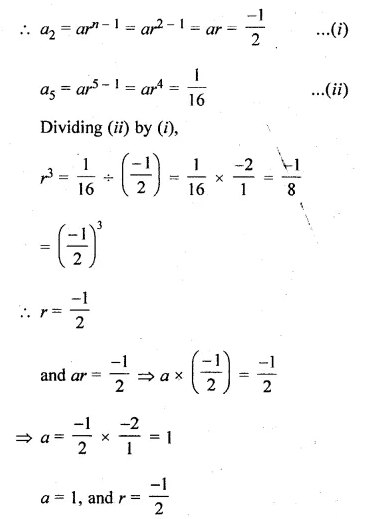Question 11.
The first term of a G.P. is 27 and 8th term is $$\\ \frac { 1 }{ 81 }$$ . Find the sum of its first 10 terms.
Solution:
In a G.P.
First term (a) = 27
a8 = 81
Let r be the common ratio, thenQuestion 12.
Find the first term of the G.P. whose common ratio is 3, last term is 486 and the sum of whose terms is 728
Solution:
Common ratio of a G.P. = 3
and last term = 486
and sum of terms = 728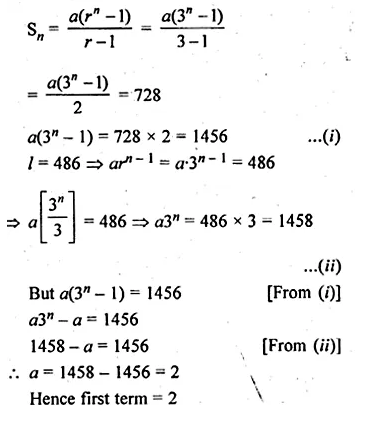Question 13.
In a G.P. the first term is 7, the last term is 448, and the sum is 889. Find the common ratio.
Solution:
In a GP.
First term (a) = 7, last term (l) = 448
and sum = 889
Let r be the common ratio, thenQuestion 14.
Find the third term of a G.P. whose common ratio is 3 and the sum of whose first seven terms is 2186.
Solution:
In a G.P.
Common ratio = 3Question 15.
If the first term of a G.P. is 5 and the sum of first three terms is $$\\ \frac { 31 }{ 5 }$$, find the common ratio.
Solution:
In a G.P.
First term (a) = 5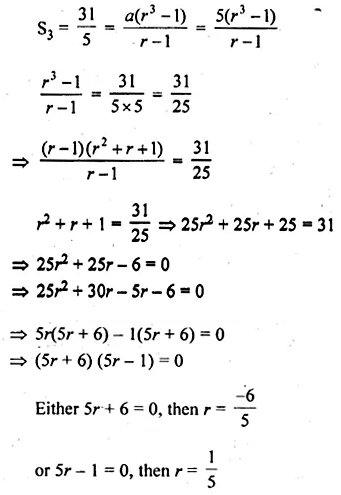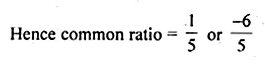Question 16.
The sum of first three terms of a GP. is to the sum of first six terms as 125 : 152. Find the common ratio of the GP.
Solution:
S3 ÷ S6 = 125 : 152
Let r be the common ratio and a be the first number, thenQuestion 17.
Find the sum of the products of the corresponding terms of the geometric progression 2, 4, 8, 16, 32 and 128, 32, 8, 2, $$\\ \frac { 1 }{ 2 }$$
Solution:
Sum of the product of corresponding terms of the G.M.sQuestion 18.
Evaluate $$\sum _{ n=1 }^{ 50 }{ \left( { 2 }^{ n }-1 \right) }$$
Solution:
$$\sum _{ n=1 }^{ 50 }{ \left( { 2 }^{ n }-1 \right) }$$
Here n = 1, 2, 3,….,50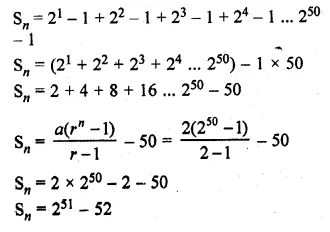Question 19.
Find the sum of n terms of a series whose mth term is 2m + 2m.
Solution:
am = 2m + 2m
a1 = 21 + 2 x 1 = 2 + 2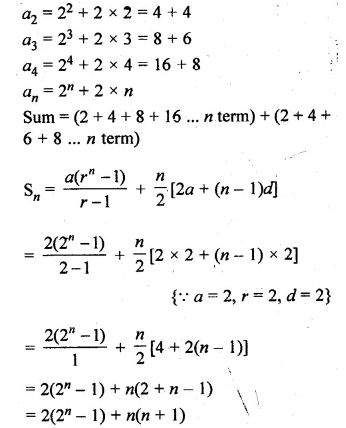Question 20.
Sum the series
x(x + y) + x2 (x2 + y2) + x3 (x3 + y3) … to n terms.
Solution:
Given
Sn = x(x + y) + x2 (x2 + y2) + x3 (x3 + y3) … n terms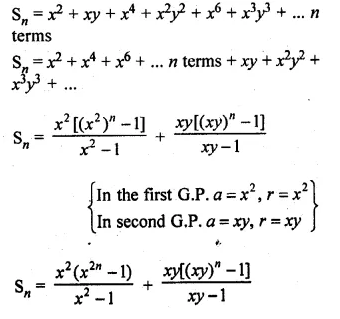Question 21.
Find the sum of the series
1 + (1 + x) + (1 + x + x2) + … to n terms, x ≠ 1.
Solution:
1 + (1 + x) + (1 + x + x2) +… n terms, x ≠ 1
Multiply and divide by (1 – x)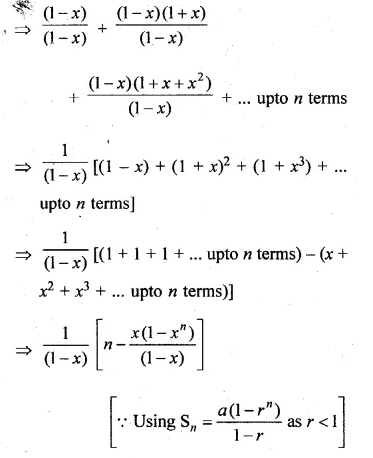Question 22.
Find the sum of the following series to n terms:
(i) 7 + 77 + 777 + …
(ii) 8 + 88 + 888 + …
(iii) 0.5 + 0.55 + 0.555 + …
Solution:
(i) 7 + 77 + 777 + … n terms
= 7[1 + 11 + 111 + … n terms]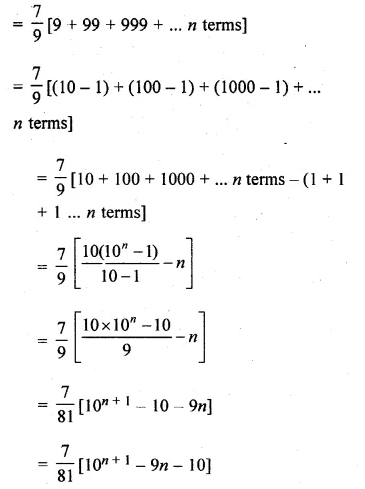Hope given ML Aggarwal Class 10 Solutions for ICSE Maths Chapter 9 Arithmetic and Geometric Progressions Ex 9.5 are helpful to complete your math homework.

If you have any doubts, please comment below. Learn Insta try to provide online math tutoring for you.